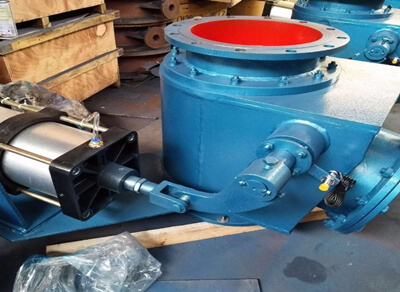﻿ Q648MX偏置式气动钟阀_温州不锈钢阀门生产厂家

13587667751Q Q：578746774# Q648MX偏置式气动钟阀

Q648MX偏置式气动钟阀使用寿命长、动作灵敏、可靠等特点。球面偏置式气动钟阀具有轴承不进灰、密封性能好、使用寿命长、动作灵敏、可靠等特点。是高炉煤系统中喷吹罐和中间罐等

## Q648MX偏置式气动钟阀

```一、简介：

Q648MX偏置式气动钟阀使用寿命长、动作灵敏、可靠等特点。球面偏置式气动钟阀具有轴承不进灰、密封性能好、使用寿命长、动作灵敏、可靠等特点。是高炉煤系统中喷吹罐和中间罐等设备上截流和密封用的理想设备。气动钟阀采用上装式结构，在高压、大口径条件下减少了阀体自身的连接螺栓，增强了阀门的可靠性且能克服系统自重对阀门正常工作的影响。

 `型号 ` `公称压力Mpa ` `壳体试验Mpa ` `供气压力Mpa ` `动作时间 ` `适用温度℃ ` `适用介质 ` `Q648MX-10` `1.0` `1.5` `0.4-0.6` `≤8S` `≤150` `煤粉等` `Q648MX-16` `1.6` `2.4`
```

 `零件名称` `阀体/阀盖` `阀瓣 ` `阀杆 ` `阀杆螺母 ` `填料 ` `垫片 ` `材料` `CF8` `F304` `F304` `QAL-4` `PRFE` `PRFE` `铜合金` `F304` `F304` `QAL-4` `PRFE` `PRFE`
```

 `公称压力PN` `公称通径DN` `D ` `D1 ` `D2 ` `b ` `f ` `n-φd ` `L` `L1` `L2` `H` `管接头` `气缸型号` `1.0Mpa` `300` `440` `400` `370` `28` `3` `12-23` `375` `485` `648` `850` `M18×1.5` `(B)QGA200×250` `400` `565` `515` `482` `32` `3` `16-26` `477` `630` `786` `1000` `M27×2` `(B)QGA250×340` `500` `675` `620` `585` `34` `3` `20-25` `625` `770` `875` `1200` `M27×2` `(B)QGA300×460` `1.6Mpa` `300` `460` `410` `378` `34` `3` `12-25` `375` `485` `648` `850` `M18×1.5` `(B)QGA200×250` `400` `580` `525` `485` `40` `5` `16-30` `477` `630` `786` `1000` `M27×2` `(B)QGA250×340` `500` `705` `650` `608` `46` `5` `20-34` `625` `770` `875` `1200` `M27×2` ```(B)QGA300×460 ```
```

 `品牌:` `用途:` `公称通径:` `百佳自控` `型号:` `Q648MX` `放料` `材质:` `铸钢` `齐全` `结构形式:` `其他` `气动` `适用介质:` `物料` `法兰` `通道位置:` `二通式` `填料密封` `压力环境:` `常压` `常温` `流动方向:` `双向` `大型` `适用范围:` `水`

#### 相关阀门产品

Q Q：578746774* 联系人
* 联系电话

QQ
* 产品名称
* 采购意向